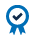## Equity Index Notional Value and Price Fluctuation

Every Equity Index futures product has its own notional, or financial, value. For Equity Index Futures, the notional value is based on the futures index level and a fixed multiplier.

## Calculating Notional Value

For Equity Index products, the notional value is defined as the product’s monetary equivalent at that price. This calculation may be expressed as follows:

Futures contract price x multiplier = Notional value

The math for calculating notional value is the same for all Equity Index futures, but the multiplier will change with each contract.

Below are a few examples;

The E-mini S&P 500® futures contract has a fixed multiplier of \$50 per contract.

If the E-mini S&P 500® futures contract trades at 2175.00, then its notional value would be \$108,750 per contract.

2175.00 x \$50 = \$108,750

The E-mini NASDAQ-100 futures contract uses a \$20 multiplier.

If the E-mini NASDAQ-100 futures contract trades at 4625.00, then the notional value would be \$92,500 per contract.

4625.00 x \$20 = \$92,500

## Minimum Price Fluctuation (Tick)

Every Equity Index futures contract has a standardized minimum price fluctuation, also known as a “tick”. The tick value is the minimum price movement, either up or down, that can occur with each futures contract.

For example, E-mini S&P500® futures contracts have a minimum price fluctuation of a quarter of an index point or 0.25. Since a full point move in the E-mini S&P500® futures contract is worth \$50, then a quarter point move would be worth \$12.50.

## Summary

Understanding the notional value of an Equity Index futures contract and the minimum price fluctuation gives traders the information they need to identify financial value for each contract and measure the cost for each tick movement in price.

For more information on the multiplier and minimum price fluctuation, please visit the product specification pages at cmegroup.com.

#### ACCREDITED COURSEDid you know that CME Institute classes can fulfill CFA and GARP continuing education requirements? Every CME Institute course can be self-reported in your CFA online CE tracker and select classes can be used for GARP credits. See which of our classes qualify for GARP credits here.